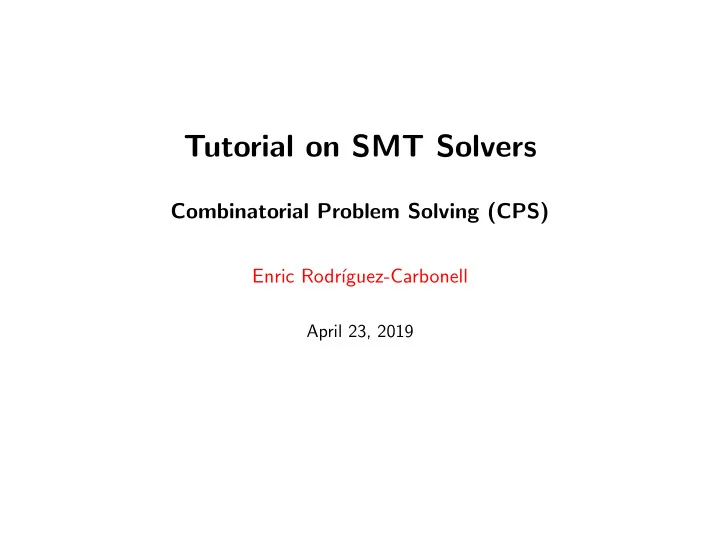# Tutorial on SMT Solvers Combinatorial Problem Solving (CPS) Enric - PowerPoint PPT Presentation

## Tutorial on SMT Solvers Combinatorial Problem Solving (CPS) Enric Rodr guez-Carbonell April 23, 2019 SMT Solvers SMT solvers take as input a (quantifier-free) first-order logic formula F over a background theory T , and return: sat (+

1. Tutorial on SMT Solvers Combinatorial Problem Solving (CPS) Enric Rodr´ ıguez-Carbonell April 23, 2019

2. SMT Solvers SMT solvers take as input a (quantifier-free) first-order logic formula F ■ over a background theory T , and return: sat (+ model): if F is satisfiable ◆ unsat : if F is unsatisfiable ◆ We will be using Z3 : http://z3.codeplex.com ■ (developed by L. de Moura and N. Bjorner at Microsoft Research) Usage: z3 [ <options> ] <input> ■ Some options: ■ -stm2 : use parser for SMT-LIB 2 input format ◆ -st : display statistics ◆ -rs:<seed> : set random seed ◆ -h : help, shows all options ◆ 2 / 10

3. Input Format: SMT-LIB 2 We will be using a small subset of this language. ■ For going beyond: Tutorial (standard version 2.0): ■ http://smtlib.github.io/jSMTLIB/SMTLIBTutorial.pdf Full standard (standard version 2.5): ■ http://smtlib.cs.uiowa.edu/papers/smt-lib-reference-v2.5-r2015-06-28.pdf 3 / 10

4. Input Format: SMT-LIB 2 First, directives. E.g., asking models to be reported: ■ (set -option :produce -models true) Second, set background theory: ■ (set -logic QF_LIA ) Standard theories of interest to us: ■ QF_LRA : quantifier-free linear real arithmetic ◆ QF_LIA : quantifier-free linear integer arithmetic ◆ QF_RDL : quantifier-free real difference logic ◆ QF_IDL : quantifier-free integer difference logic ◆ SMT-LIB 2 does not allow to have mixed problems ■ (although some solvers support it outside the standard) 4 / 10

5. Input Format: SMT-LIB 2 Third, declare variables. ■ E.g., integer variable x : (declare -fun x () Int) E.g., real variable z 1 3 : (declare -fun z_1_3 () Real ) 5 / 10

6. Input Format: SMT-LIB 2 Fourth, assert formula. ■ Expressions should be written in prefix form: ■ ( < operator > < arg 1 > ... < arg n > ) (assert (and (or (<= (+ x 3) (* 2 u) ) (>= (+ v 4) y) (>= (+ x y z ) 2) ) (= 7 (+ (ite (and (<= x 2 ) (<= 2 (+ x 3 (- 1)))) 3 0) (ite (and (<= u 2 ) (<= 2 (+ u 3 (- 1)))) 4 0) ) ) ) ) 6 / 10

7. Input Format: SMT-LIB 2 and , or , + have arbitrary arity ■ - is unary or binary ■ * is binary ■ ite is the if-then-else operator (like ? in C, C++, Java). ■ Let a be Boolean and b , c have the same sort S . Then (ite a b c) is the expression of sort S equal to: b if a holds ◆ c if a does not hold ◆ 7 / 10

8. Input Format: SMT-LIB 2 Finally ask the SMT solver to check satisfiability ... ■ (check -sat) ... and report the model ■ (get -model) Anything following a ; up to an end-of-line is a comment ■ 8 / 10

9. Input Format: SMT-LIB 2 (set -option :produce -models true) (set -logic QF_LIA ) (declare -fun x () Int) (declare -fun y () Int) (declare -fun z () Int) ; This is an example (declare -fun u () Int) (declare -fun v () Int) (assert (and (or (<= (+ x 3) (* 2 y) ) (>= (+ x 4) z) ) ) ) (check -sat) (get -model) 9 / 10

10. Output Format 1st line is sat or unsat ■ If satisfiable, then comes a description of the solution in a model ■ expression, where the value of each variable is given by: ( define − fun < variable > () < sort > < value > ) Example: ■ sat (model (define -fun y () Int 0) (define -fun x () Int (- 3)) (define -fun z () Int 2) ) 10 / 10

More recommend